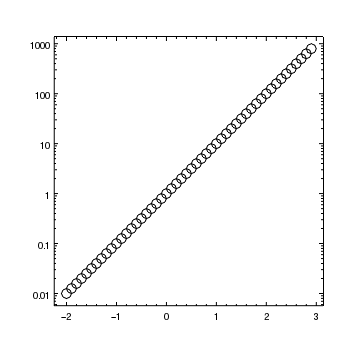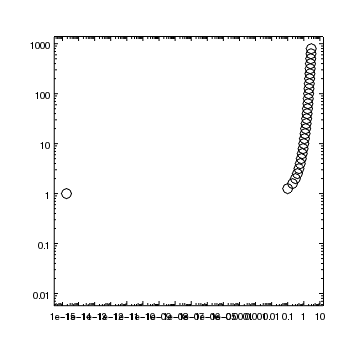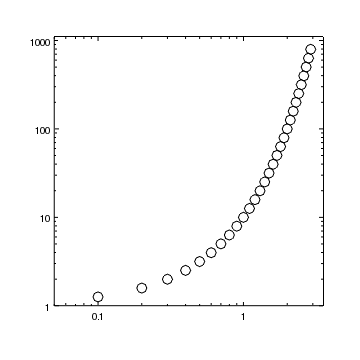## How do I change an axis to use a logarithmic scale?

The log_scale and lin_scale commands change an axis to be drawn using a logarithmic or linear scale, respectively (the longer forms logarithmic_scale() and linear_scale() can also be used).

chips> clear()
chips> x = np.arange(-2, 3, 0.1)
chips> y = 10**x
chips> set_curve(["line.style", "noline", "symbol.style", "circle", "symbol.fill", False])
chips> log_scale(Y_AXIS)### What happens to negative values?

ChIPS will allow you to convert an axis to logarithmic scale even if it displays zero or negative values; the minimum positive value of the data will be used to determine the new limits if required. With the plot from above, the minimum positive value should be 0.1 but due to numerical artifacts it is actually 1e-15, which can be seen by converting the X-axis to a logarithmic scale:

chips> x[np.where(x > 0)].min()
1.7763568394002505e-15
chips> log_scale(X_AXIS)We can adjust the limits on both axes to avoid displaying this point; for example

chips> limits(X_AXIS, 0.05, AUTO)
chips> limits(Y_AXIS, 1, AUTO)#### The ChIPS GUI

The ChIPS GUI makes it easy to modify a visualization using your mouse, rather than Python functions. The GUI can also be used to add annotations - such as labels, lines, points and regions - and to zoom or pan into plots.